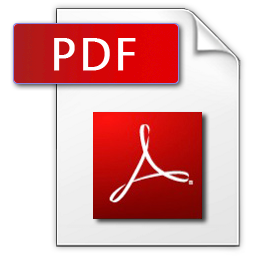﻿ Вісник Тернопільського національного технічного університету
﻿﻿

### Simulation of dynamic modes in the asynchronous motor

 Назва Simulation of dynamic modes in the asynchronous motor Назва англійською Simulation of dynamic modes in the asynchronous motor Автори Viktor Lyshuk (https://orcid.org/0000-0003-4049-8467); Yosyp Selepyna (https://orcid.org/0000-0002-2421-1844); Sergiy Kostiuchko (https://orcid.org/0000-0002-1262-6268); Sergiy Litkovets (https://orcid.org/0000-0003-2896-8518) Принадлежність Lutsk National Technical University, Lutsk, Ukraine; National University of Water and Environmental Engineering, Rivne, Ukraine Бібліографічний опис Simulation of dynamic modes in the asynchronous motor / Viktor Lyshuk; Yosyp Selepyna; Sergiy Kostiuchko; Sergiy Litkovets / Scientific Journal of TNTU. — Tern. : TNTU, 2019. — Vol 94. — No 2. — P. 104–110. — (Mathematical modeling. Mathematics) Bibliographic description: Lyshuk V.; Selepyna Y.; Kostiuchko S.; Litkovets S. (2019) Simulation of dynamic modes in the asynchronous motor. Scientific Journal of TNTU (Tern.), vol. 94, no 2, pp. 104-110. DOI: https://doi.org/10.33108/visnyk_tntu2019.02.104 УДК 519.876.5; 621.313.33 Ключові слова differential equations, mathematical model, asynchronous motor, numerical methods. The mathematical model of asynchronous three-phase motor, which takes into account the saturation of the magnetic system, is constructed in this paper. The system of differential equations of the electromagnetic state is formed in the normal Cauchy form. This form of equations presented in the matrix form is very convenient for numerical integration, since it is implemented by explicit methods that are simpler in computer realization than implicit ones. This approach makes it possible to describe the complex physical processes in the motors, namely the effect of saturation of the magnetic circuit and mechanical rotational motion. The use of the proposed model of the asynchronous motor, created by its real passport data, provides an opportunity for adequate real-time modeling. FORTRAN programming language and graphic editor GRAPHER are used to calculate dynamic regimes. The explicit Euler method is used for numerical integration of differential equations. The proposed model can be used to analyze the modes of operation of the motors as an autonomous element and an element of the electromechanical system. It is shown that this model corresponds to the classical theory of electric machines. ISSN: 2522-4433 Перелік літератури Vas’kovskyy Yu. M. Matematychne modeliuvannia electromekhanichnykh peretvoriuvachiv energii. Kyiv: KPI, 2003. 164 p. [Іn Ukrainian]. Kopylov I. P. Matematicheskoje modelirovanije electricheskikh mashin. Moscow: Vysshaja shkola Publ., 2001. 327 p. [Іn Russian]. Lyshuk V. V., Selepyna Y. R. Matematychne modeliuvannia dynamichnykh rezhymiv hlybokopaznykh asynkhronnykh dvyhuniv. Visnyk TNTU imeni I. Puliuja. 2014. No.4 (76). P. 210–223. [In Ukrainian]. Ong Chee-Mun. Dynamic Simulation of Electric Mashinery. New Jersej, 1998. 626 p. Syvokobylenko V. F., Vasylets’ S. V. Matematychna model’ asynkhronnoho dvyhuna z urakhuvanniam nasychennia stali ta vytisnennia strumu v rotori. Modeliuvannia ta informatsiyni tekhnolohii. 2013. No. 69. P. 3–10. [In Ukrainian]. Chaban V. Chysel’ni metody. L’viv: L’vivs’ka politekhnika, 2001. 186 p. [Іn Ukrainian]. Chaban V. Electromahnitni protsesy. L’viv: Prostir M, 2017. 412 p. [Іn Ukrainian]. References: The mathematical model of asynchronous three-phase motor, which takes into account the saturation of the magnetic system, is constructed in this paper. The system of differential equations of the electromagnetic state is formed in the normal Cauchy form. This form of equations presented in the matrix form is very convenient for numerical integration, since it is implemented by explicit methods that are simpler in computer realization than implicit ones. This approach makes it possible to describe the complex physical processes in the motors, namely the effect of saturation of the magnetic circuit and mechanical rotational motion. The use of the proposed model of the asynchronous motor, created by its real passport data, provides an opportunity for adequate real-time modeling. FORTRAN programming language and graphic editor GRAPHER are used to calculate dynamic regimes. The explicit Euler method is used for numerical integration of differential equations. The proposed model can be used to analyze the modes of operation of the motors as an autonomous element and an element of the electromechanical system. It is shown that this model corresponds to the classical theory of electric machines. Завантажити﻿
Всі права захищено © 2019. Тернопільський національний технічний університет імені Івана Пулюя.